Metals can emit electrons when they are supplied with sufficient energy. In thermionic emission this energy is supplied by heating the wire with an electric current. In the case of photoelectricity the energy is supplied by illuminating the surface of the metal with ultra violet light. This was first discovered by Heinrich Hertz during his work on radio waves but he did not follow up his discovery.

Further investigations showed that other metals can emit photoelectrons. Photoelectrons are identical to any other electron, the prefix ‘photo’ just indicates how the electron gained its energy. Measurements on the photoelectric effect showed that;

·         the number of photoelectrons emitted per second from any metal is proportional to the intensity of the illumination.

·         photoelectrons emitted from a metal have a range of kinetic energies up to a maximum value.

·         no photoelectrons are emitted if the frequency of the incident light fall below a certain threshold frequency irrespective of how intense the light is.

## The failure of classical wave theory to explain photoelectric emission

The existence of a threshold frequency presented a great puzzle to scientists of the time. According to wave theory there should not be a threshold frequency at all. Each electron should take energy from each incident light wave until it gained sufficient to leave the metal surface. With low frequency light emission should take longer to occur but it should eventually happen. It doesn’t !

## The significance of Einstein’s explanation of photoelectricity

Einstein’s theory was developed from the quantum theory put forward a few years earlier by Max Planck to explain the black body radiation curves (see earlier). In 1905 Einstein extended Planck’s ideas and used them to explain the existence of the threshold frequency in the photoelectric effect.

He suggested that the quantum of energy emitted by an atom continued to exist as a concentrated packet of energy. So when an atom emits light it loses a ‘lump’ of energy carried away by a wavepacket, which we call a photon. The idea of a wavepacket helps to visualise the idea of a photon which is different to waves on water. A wavepacket is a concentration of energy that travels away from the source in one direction only. A source of light emits photons travelling in all directions but each photon travels away only in one specific direction.

The energy of a photon is proportional to its frequency and is given by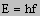where     h = Planck’s constant

f = frequency

If one considers a stream of light directed at a metal surface. Any free electron near the surface of the metal could be struck by a photon and thus gain kinetic energy. If sufficient energy is gained the electron may leave the metal surface. The energy gained must be equal to the whole energy of the photon (hf).

To escape from the metal the electron must do work. The electron can only escape if it gains sufficient energy from an encounter with a single photon. Thus the photon energy must be greater than the work needed to escape from the metal surface and thus the maximum KE of the photoelectron will be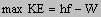or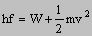where     W = work function of the metal (the work necessary to remove an electron from the metal)

This equation is known as Einstein’s photoelectric equation.

### Some things to consider

1.       Why do the emitted photoelectrons have a range of KE’s even if monochromatic light is used ?

2.       Show that the threshold frequency is given by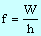3.       What would happen to the photoelectric emission if the metal is at

a)      a positive potential

b)      a negative potential

#### Millikan’s photoelectric experiment

Einstein’s theory was proven by Millikan’s experiments in 1916. If a positive voltage is applied to the metal then it will stop photoelectric emission at a particular frequency when the voltage reaches a value known as the stopping voltage Vs. Extra work equal to eVs has to be done to remove the electron from the metal and thus photoelectric emission will stop when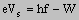Einstein predicted that a graph of Vs against frequency would have the same gradient irrespective of the material used.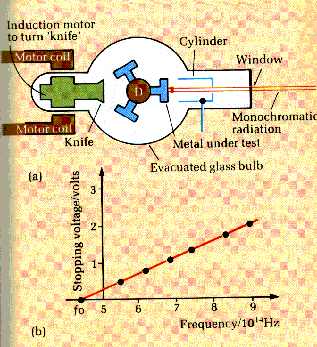Millikan devised a vacuum tube in which clean metal surfaces could be cut. He used a drum with three different metals. The drum could be rotated and a freshly prepared surface could be obtained using a knife which could also be rotated using an induction motor arrangement. Light of a selected frequency could be obtained from a spectrometer and shone onto the prepared metal surface. A metal cylinder collected any photoelectrons released from the metal. An electroscope was used to show the charge collected by the cylinder. Millikan then increased the potential of the metal surface until the electroscope showed that no photoelectrons were being emitted. This potential was then equal to the stopping potential for that frequency of light.

Millikan made a series of measurements for each metal over a range of different frequencies. The results he obtained fitted Einstein’s predictions exactly and gave a value for h of 6.6 x 10-34 Js.

The main features of the graph are

·         the gradient for any metal is always equal to h/e

·         the intercept on the f axis is equal to f0, the threshold frequency of the metal, hence the work function of the metal may be calculated.

next